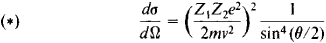# Rutherford's Formula

## Rutherford’s Formula

a formula for the effective scattering cross section of nonrelativistic charged point particles interacting according to Coulomb’s law. It was derived in 1911 by E. Rutherford.

In a center-of-mass system, that is, a system in which the total momentum of the colliding particles is equal to zero, Rutherford’s formula has the formwhere dσ/dΩ is the scattering cross section into a unit solid angle, θ is the scattering angle, m = m1m2/(m1 + m2) is the reduced mass (m1 and m2 are the masses of the colliding particles), v is the relative velocity (the difference in the velocities of the particles), Z1e and Z2e are the electric charges of the particles, and e is the elementary electric charge. Rutherford’s formula is valid in both classical and quantum theory.

Formula (*) was used by Rutherford in interpreting experiments on the scattering of alpha particles at large angles (θ > 90°) on passage through thin metal foils. As a result of these experiments, Rutherford concluded that virtually the entire mass of the atom is concentrated in a small positively charged nucleus. This discovery formed the basis of modern conceptions of atomic structure.

### REFERENCES

Landau, L. D., and E. M. Lifshits. Mekhanika, 3rd ed. Moscow, 1973. (Teoreticheskaia fizika, vol. 1.)
Landau, L. D., and E. M. Lifshits. Kvantovaia mekhanika. 2nd ed. Moscow, 1963. (Teoreticheskaia fizika, vol. 3.)

S. M. BILEN’KII

Site: Follow: Share:
Open / Close